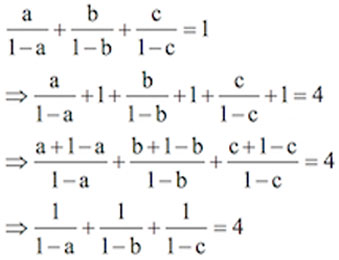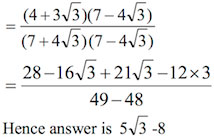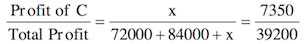# Sample Aptitude Questions of KPMG

1. The total cost of 8 buckets and 5 mugs is Rs. 92 and the total cost of 5 buckets and 8 mugs is Rs. 77. Find the cost of 2 mugs and 3 buckets. A.
1. Rs. 35
2. Rs. 70
3. Rs. 30
4. Rs. 38

CP of 1 bucket = Rs. X
CP of 1 mug = Rs. Y
∴ 8x + 5y = 92………….. (i)
5x + 8y = 77 …………….(ii)
By equation (i) × 5 – equation (ii) × 8.
40x + 25y – 40x – 64y
= 460 – 616 ⇒ − 39y = - 156⇒ y = 4
From equation (i),
8x + 20 = 92 ⇒8x = 92 – 20 = 72 ⇒ x = 9
∴ CP of 2 mugs and 3 buckets
= 2 × 4 + 3 × 9 = 8 + 27 = Rs. 35
2. If a/(1-a) + b/(1-b) + c/(1-c) = 1 then the value of 1/(1-a) + 1/(1-b) + 1/(1-c) is:
1. 1
2. 3
3. 4
4. 01. If (x – 3)2 + (y – 5)2 + (z – 4)2 = 0, then the value of x2/9 + y2/25 + z2/16 is:
1. 12
2. 9
3. 3
4. 1

(x – 3)2 + (y – 5)2 + (z – 4)2 = 0
⇒ x – 3 = 0 ⇒ x= 3
Y – 5 = 0 ⇒ y = 5
Z – 4 = 0 ⇒ z = 4
x2 / 9+ y2 / 25+ z2 /16 = 9/9 + 25/25 + 16/16 =1+1+1 = 3
2. If 4x/3 + 2P = 12 for what value of P, x = 6?
1. 6
2. 4
3.
4. 1

When x = 6, (4 * 6)/3 + 2P = 12
⇒ 8 + 2P = 12
⇒ 2P = 12 – 8 = 4
⇒ P = 2
3. The value of (4+3√3)/(7+4√3) is:
1. 5√3 - 8
2. 5√3 + 8
3. 8√3 + 5
4. 8√3 - 5

Expression = (4+3√3)/(7+4√3)
Rationalizing the denominator4. A & B started a business together by investing Rs. 36000/- and Rs. 42000/- respectively. Both of them invested for one year whereas after 6 months from the start C joined them by investing a certain amount. If they earned an annual profit of Rs. 39,200/- out of which C’s share is Rs. 7350/-. What is the investment of C?
1. Rs. 24000/-
2. Rs. 36000/-
3. Rs. 27000/-
4. Rs. 33000/-
5. Rs. 30000/-

Let the investment of C=Rs x
Ratio of profits of A &B &C
=36000×12 : 42000×12 : x×6
=72000:84000: xSolving, x = 36000. B option.
5. What are the marks obtained by Harish in Maths ?
I. Average marks obtained by Harish in Maths and economies are 83.
II. Average marks obtained by Harish in Maths and statistics are 92.
1. The data in statement I alone are sufficient to answer the question, while the data in statement II alone not sufficient to answer the question.
2. The data in both the statements I & II together are necessary to answer the question.
3. The data either in statement I alone or in statement II are sufficient to answer the question.
4. The data in both the statements I & II together are insufficient to answer the question.
5. The data in statement II alone are sufficient to answer the question, while the data in statement I alone are not sufficient to answer the question.

We cannot find the marks in maths alone even by using both the statements together as average marks will not tell us exact marks in Maths.
Data is insufficient. Hence D option.
6. By what percent is Rajesh’s salary more/less than Mangesh’s ?
I. Salary of Rajesh is Rs. 12000/-.
II. Salary of Rajesh and Mangesh together is Rs 28000/-.
1. The data in statement I alone are sufficient to answer the question, while the data in statement II alone not sufficient to answer the question.
2. The data in both the statements I & II together are necessary to answer the question.
3. The data either in statement I alone or in statement II are sufficient to answer the question.
4. The data in both the statements I & II together are insufficient to answer the question.
5. The data in statement II alone are sufficient to answer the question, while the data in statement I alone are not sufficient to answer the question.

By using both the statements together we can find the salary of Mangesh . Hence we can find the percentage change between the salaries of Rajesh and Mangesh. B option.
7. What is the strength of MBA institute where students study only HR, Marketing and Finance ?
I. Number of students studying HR, Marketing and Financial are in the ratio of 2 : 3: 5 respectively.
II. Number of students studying Marketing is more than those studying HR by 800.
1. The data in statement I alone are sufficient to answer the question, while the data in statement II alone are not sufficient to answer the question.
2. The data either in statement I alone or in statement II alone are sufficient to answer the question.
3. The data in both the statements I & II together are necessary to answer the question.
4. The data in statement II alone are sufficient to answer the question, while the data in statement I alone not sufficient to answer the question.
5. The data in both the statements I & II together are insufficient to answer the question.

Using both statements together , Let no. of students of HR, marketing and Finance be 2x,3x,5x and also 3x-2x=800
x=800
So total students =2x+3x+5x=10×800=8000. Hence C.
8. A shopkeeper purchased 96 identical shirts @ Rs. 220/- each. He spent Rs. 3800/- on transport and packing and fixed a marked price of Rs. 450/- each shirt. However, he decided to give discount of 20% on the marked price of each shirt. If he could sell all the shirts, what is the approximate percent profit earned by him?
1. 44
2. 39
3. 33
4. 31
5. 30